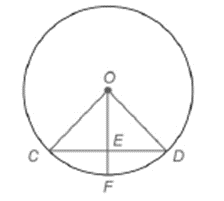Chapter 6.3, Problem 1EElementary Geometry For College St...

7th Edition
Alexander + 2 others
ISBN: 9781337614085

Solutions

Chapter
SectionElementary Geometry For College St...

7th Edition
Alexander + 2 others
ISBN: 9781337614085
Textbook Problem

Note: Exercises preceded by an asterisk are of a more challenging nature. G i v e n :               ⊙ O     w i t h O E ¯ ⊥ C D ¯                                                             C D = O C F i n d :                     m C F ⌢To determine

To find:

To find mCF^.

Explanation

Given that O with OE¯CD¯ and CD=OC

Consider the following figure,

From the above figure shows that OC=OD. Since radius of O is same for all points on circle.

From the given condition CD=OC=OD

Therefore, ΔOCD is equilateral triangle. Therefore, C=COD=D=60

Let us take ΔOCE,ΔOED

Also, OEC=OED=90. Since OE¯CD¯.

Theorem:

If a line is drawn through center of a circle perpendicular to a chord then it bisects the chord and its arc

Still sussing out bartleby?

Check out a sample textbook solution.

See a sample solution

The Solution to Your Study Problems

Bartleby provides explanations to thousands of textbook problems written by our experts, many with advanced degrees!

Get Started

Find more solutions based on key concepts